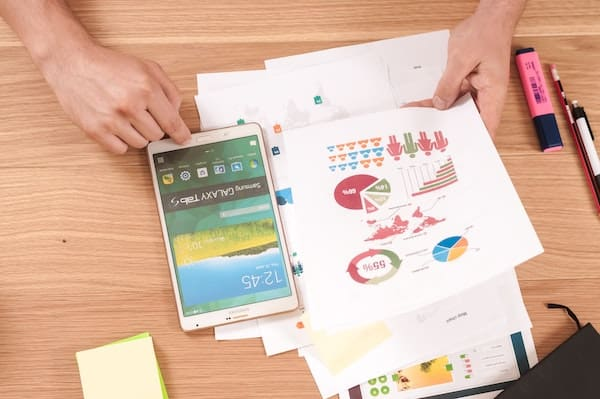# Financial Terms “P”## Plant assets

Plant assets are noncurrent physical assets used in business operations. Plant assets include land and buildings. Plant assets are also called fixed assets. The category is sometimes shown on the balance sheet as property, plant, and equipment or PP&E.

## Present value

Present value is a time value of money (TVM) concept. It is a lump sum of money today or at the beginning of a TVM problem. It is the current discounted value of all future cash flows. Discounting is the process of bringing money to the present. It is the opposite of compounding which is growing money to the future. Financial calculators and Excel use the PV function to calculate present value.

The present value formula includes the following variables: FV = future value, i = periodic interest rate, and n = number of periods.

Assume you will receive \$1,331 at the end of three years. If you require a 10% return, what is the present value today? The present value is \$1,000. See the following example.

## Price-earnings ratio

The price-earnings or P/E ratio is a financial ratio where the market price is divided by earnings. It is one of the profitability ratios.

## Profit and loss statement

The profit and loss (P&L) statement is also called the income statement. The format of the income statement is revenues minus expenses.

## Profit margin

A profit margin is a ratio of profit to sales. A higher profit margin is more desirable.

1. net profit margin is net income or net profit divided by sales
2. gross profit margin is gross profit divided by sales

Jeff Mankin

Jeff Mankin teaches financial literacy. His website is FinallyLearn.com.